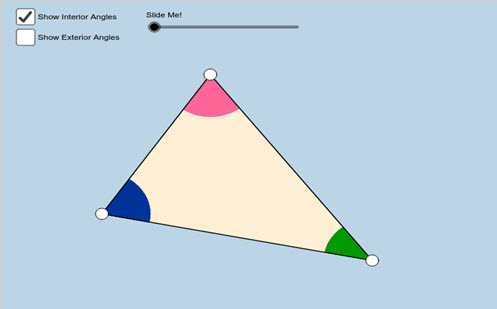Go to website

# Polygon angle theorems

This GeoGebra applet interactive allows students to explore the angle sums of polygons by finding the triangles inside the polygon.

Year level(s) Year 7
Audience Teacher
Purpose Teaching resource
Format Interactive
Teaching strategies and pedagogical approaches Explicit teaching
Keywords angle sum, polygons

## Curriculum alignment

Strand and focus Space
Topics Angles and geometric reasoning
AC: Mathematics (V9.0) content descriptions
AC9M7M04
Identify corresponding, alternate and co-interior relationships between angles formed when parallel lines are crossed by a transversal; use them to solve problems and explain reasons

AC9M7M05

Demonstrate that the interior angle sum of a triangle in the plane is 180° and apply this to determine the interior angle sum of other shapes and the size of unknown angles

Numeracy progression Understanding geometric properties (P6)